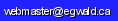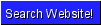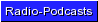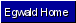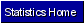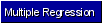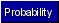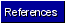Egwald Statistics: Econometrics, Probability, and Stochastic Processes by Elmer G. Wiens Egwald's popular web pages are provided without cost to users. Please show your support by joining Egwald Web Services as a Facebook Fan:Follow Elmer Wiens on Twitter:Run regressions online. Easy to learn. Easy to use.   a. Linear multiple regression.       1. Example: Predicting Canadian Elections using the equation:             C = ß0 + ß1 * E + ß2 * OQ + ß3 * P + ß4 * BC                 where             C = Percent of seats of Canada taken by the winning party             E = Percent of seats of Eastern Canada taken by the winning party             OQ = Percent of seats of Ontario and Quebec taken by the winning party             P = Percent of seats of Prairie Provinces taken by the winning party             BC = Percent of seats of B.C. taken by the winning party       Regression equation using the data for 1949-2004: Predicting Canadian Elections. These 18 elections yield the equation:             C = -17.321 + 0.13 * E + 0.857 * OQ + 0.117 * P + 0.113 * BC               (Each regression coefficient is statistically significant)       2. Do your own regression study.   b. Restricted linear regression.       1. Example: Cobb Douglas Production Function       2. Do your own restricted regression study Check out the derivation of the regression model. Statistics, multiple regression, and numerical analysis references. Learn key concepts used in statistics and regression analysis.   a. Probability       1. Graphs of the normal and gamma probability distributions.       2. Graphs of the chi-square, Student-t, and F probability distributions.       3. Graphs of the sample mean and variance distributions.       4. Hypotheses testing of means and variances.   b. Stochastic Processes       1. Random Walk. C. Background and References.       1. Linear Algebra background to least squares (multiple regression).       2. Statistics and probability theory references. Links The Math Help Forum. https://mathhelpforum.com/community. Study econometrics in the Department of Economics of the KU Leuven, Belgium.Copyright © Elmer G. Wiens:   Egwald Web ServicesAll Rights Reserved.    Inquiries